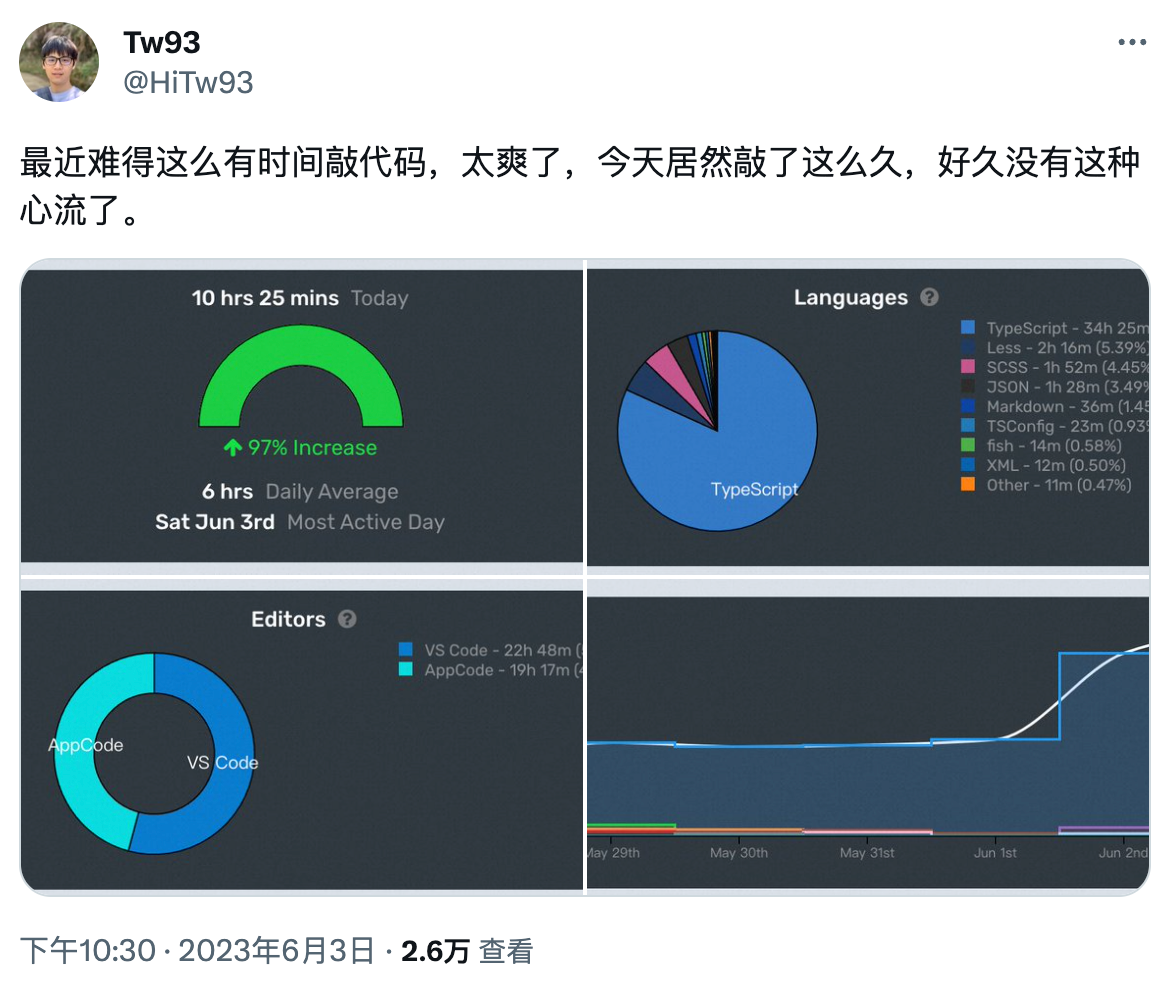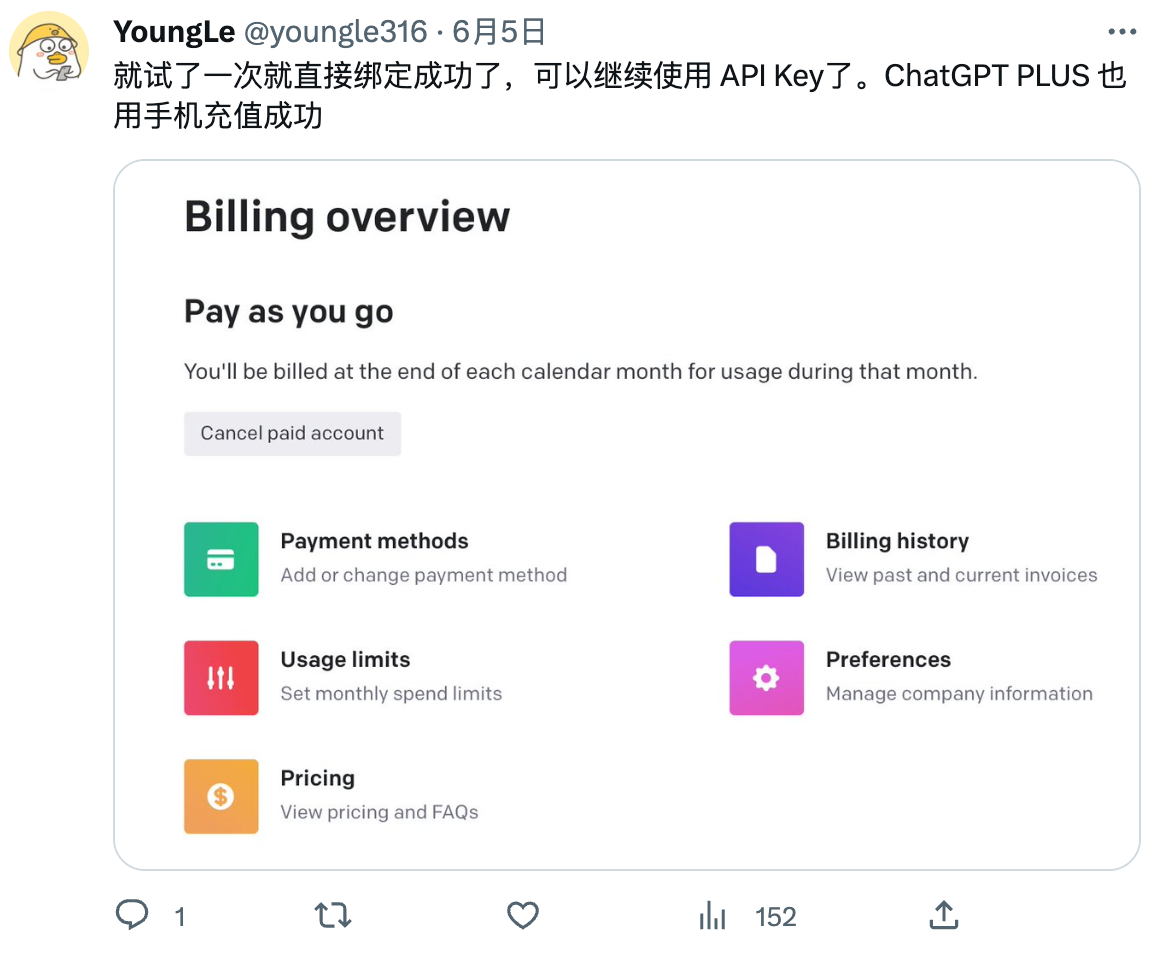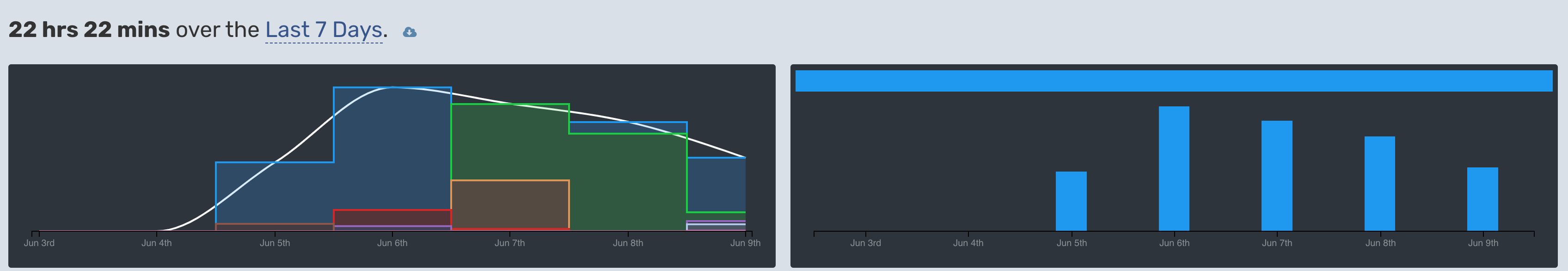weekly
2023-06-09

# Weekly 08 - 趁手的兵器self.__wrap_b=(t,n,e)=>{e=e||document.querySelector(`[data-br="\${t}"]`);let a=e.parentElement,r=R=>e.style.maxWidth=R+"px";e.style.maxWidth="";let o=a.clientWidth,c=a.clientHeight,i=o/2-.25,l=o+.5,u;if(o){for(;i+1<l;)u=Math.round((i+l)/2),r(u),a.clientHeight===c?l=u:i=u;r(l*n+o*(1-n))}e.__wrap_o||(typeof ResizeObserver!="undefined"?(e.__wrap_o=new ResizeObserver(()=>{self.__wrap_b(0,+e.dataset.brr,e)})).observe(a): false&&0)};self.__wrap_b(":R6rdn6:",1)

2分钟阅读